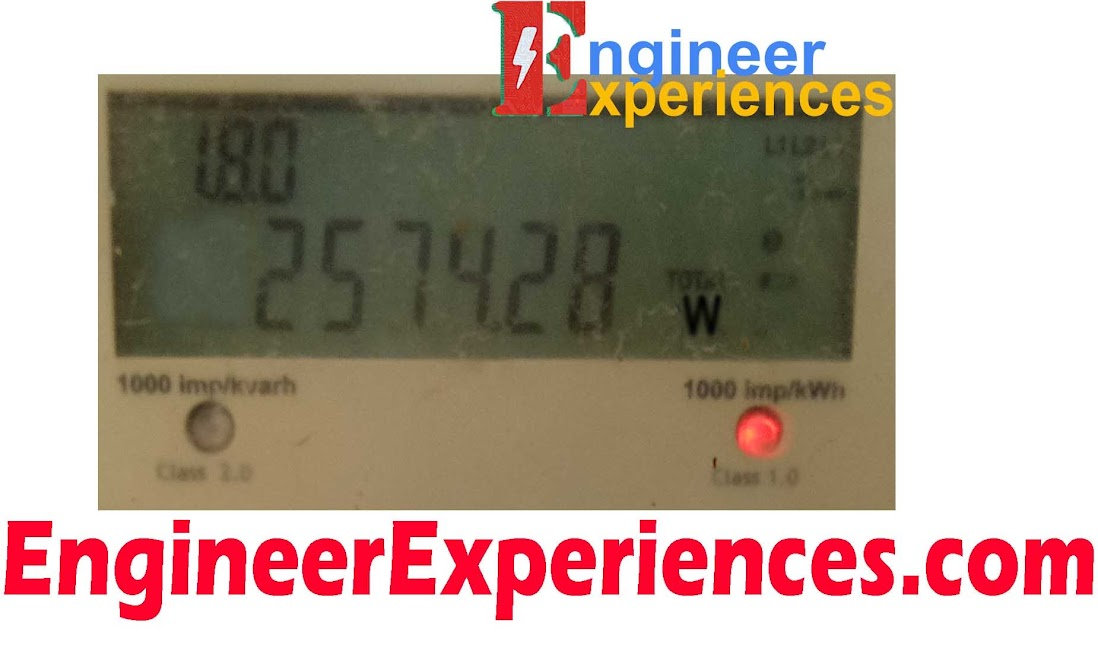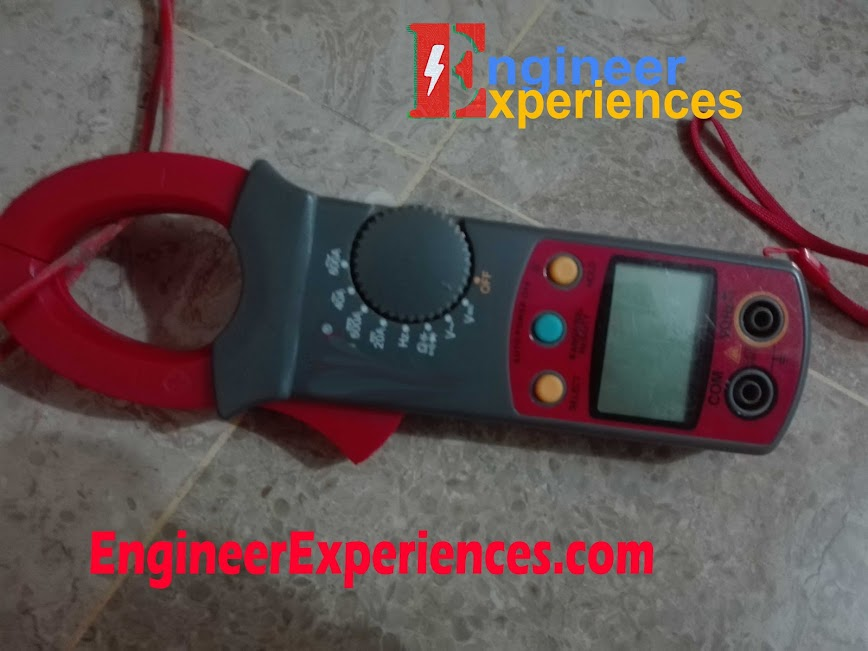# How to Check Accuracy of Energy Meters

Table of Contents

# Accuracy Checking of Energy Meters

Accuracy checking of Energy Meter is not a difficult thing. A person having a clamp meter or power analyzer can easily check the accuracy whether that person is a lay man or a techy person. There are major three methods to compare accuracy of an Energy Meter. Let’s start with the most common method.

## Accuracy Checking of Energy Meter through impulse method

This is the most common method in which you need nothing except a stop watch. In my opinion this method will not give authentic result. Nevertheless, let’s take a fast look on this method as well.

First of all take a look on this photo below:Figure 1: Instantaneous Power on Digital Energy Meter

This is a LCD of an Energy Meter which is giving instantaneous power of 2574 Watts. At the same time, you can see a LED blinking on the right side which is also indicating the power usage of 2574 watts. But how?

Open “Stop Watch” in your mobile and measure time of 10 pulses. Say it is 13.91 seconds, this is your Time observed.

i.e. To = 13.91 Seconds

Now we will calculate ideal time that should be passed during 10 samples at 2574 watts.

$Tc = \frac{3600 X No. of Impulses }{Meter Constant X Running Load (kW)}$

We have all values which are as follows:

No. of impulses which we took from stop Watch = 10

Meter Constant  = 1000 imp/kWh

Running load (Instantaneous Load) = 2574 Watts

Side Note: Meter constant is something that is always written on Energy Meters and different for different meter depends on the Energy Meter manufacturer. You can see meter constant of 1000imp/kWh on the above image of Energy Meter.

$Tc = \frac{3600 X 10 }{1000 X 2.574 (kW)}$

$Tc = 13.986 Seconds$

This Tc is time calculated which is ideal time for 2574 watts.

### Slowness or fastness of Energy Meters

Now, its time to calculate whether this Energy Meter is slow or fast.

$Slowness / Fastness = \frac{Tc - To }{To} X 100$

Here,

Tc = 13.986 Seconds
To = 13.91 Seconds
$Slowness / Fastness = \frac{13.99 - 13.91 }{13.91} X 100$
$Slowness / Fastness = 0.57 %$

As the value is positive so the Energy meter is 0.57% fast. Now we will check the class of Energy Meter.

Study article on what is the meaning of class accuracy of energy meters

This Energy Meter is of Class 1 accuracy as written on the Energy Meter present above. It means measurement can be deviated from the original value in the range of 1 %.  As, Our answer is 0.57 % So, error of Energy Meter is in acceptable range.

## Accuracy Checking of Energy Meter through Clamp Meter

In the above method, you have seen a lot of calculations but this method is fast and authentic method. Though this method give less accurate results but still this method is more acceptable than the above one.

First of all take a clamp meter and set it on AC Current measuring point. While using Clamp Meter always keep in mind that never grab both live and neutral wire inside the clamp. Clamp Meter will never give you any sensible results. Because when grab both wires, each wire cancel the effect of other one.

Grab the wire through clamp meter like the image below:Figure 2: Current Measurement through Clamp Meter

Now take reading of the clamp meter. Say the reading of meter is 11.68 Amps. Now we have to assume some values to convert it into power.

If we take example of single phase then the assumed values will be:

Voltage = 220 Volts (110 Volts in American Electric System)
CosØ = 1
Instantaneous-Power = V X I X CosØ
Instantaneous_Power = 220V X 11.68 Amps X 1
Instantaneous-Power = 2569 Watts

So, according to clamp meter Instantaneous Power should be 2569 watts but Energy Meter is showing 2574 watts. So, the difference is in acceptable range because you are taking assumptions of other important factors like voltage and power factor.

Side Note: If you measure the voltage through voltmeter present in your clamp meter, your result will get more closer to the original value but still you have to assume power factor.

## Accuracy Checking of Energy Meter through Power Analyzer

This is most accurate and fast method as compared to other methods. You just have to connect voltage terminals in parallel and clamp in series of load and you will find the instantaneous power. Compare both instantaneous powers and you will get your result. I am not going into discussion into this method because of its easiness.

If you need any more help, feel free to comment below. Also don't forget to like our facebook page. ?

#### Ismail Sarwar

Electrical engineering is my profession, my hobby and my passion. I completed Bachelors of Electrical Engineering in 2015. Currently, I am doing Masters in Electrical Systems Engineering. Power Electronics and Embedded Systems are my fields of specialization. In free time I listen music and watch movies.

## 3 thoughts to “How to Check Accuracy of Energy Meters”

1. Anonymous says:

very effective

2. Ajit Lakra says:

I liked the article on accuracy of energy meter very much.It is very useful and of very use.Can you pl explain the use of power analizer once again on how to connect voltage terminals in parallel and clamp in series of load through a diagram.

This site uses Akismet to reduce spam. Learn how your comment data is processed.